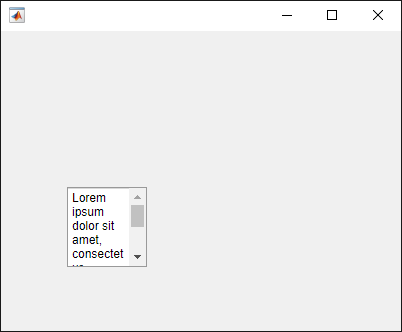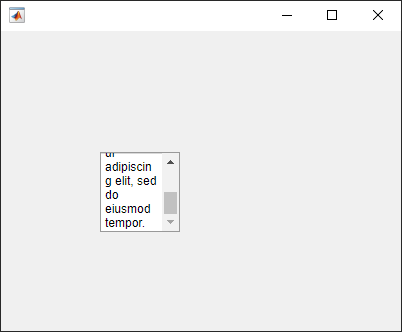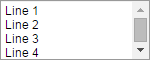# uitextarea

## 语法

``txa = uitextarea``
``txa = uitextarea(parent)``
``txa = uitextarea(___,Name,Value)``

## 说明

``txa = uitextarea` 在新图窗窗口中创建一个文本区域，并返回 `TextArea` 对象。MATLAB® 调用 `uifigure` 函数来创建该图窗。`

``txa = uitextarea(parent)` 在指定的父容器中创建文本区域。父容器可以是使用 `uifigure` 函数创建的 `Figure` 或其子容器之一。`

``txa = uitextarea(___,Name,Value)` 使用一个或多个 `Name,Value` 对组参数指定 `TextArea` 属性。可以将此选项与前面语法中的任何输入参数组合一起使用。`

## 示例

```fig = uifigure; txa = uitextarea(fig);``````fig = uifigure; txa = uitextarea(fig,... 'Value', {'First Name Last Name';... 'Address 1'; 'Address 2';'City, State'; 'Postal Code'});````size = txa.Position(3:4)`
```size = 150 60```

`txa.Position(3:4) = [155 75];````fig = uifigure; txa = uitextarea(fig); txa.Position = [100 100 80 80]; txa.Value = 'Lorem ipsum dolor sit amet, consectetur adipiscing elit, sed do eiusmod tempor.';````scroll(txa,'bottom')````function comments % Create figure window and components fig = uifigure('Position',[500 500 430 275]); label1 = uilabel(fig,... 'Position',[100 164 100 15],... 'Text','Enter Comments:'); label2 = uilabel(fig,... 'Position',[100 75 175 15],... 'Text',''); textarea = uitextarea(fig,... 'Position',[100 100 150 60],... 'ValueChangedFcn',@(textarea,event) textEntered(textarea, label2)); % Create ValueChangedFcn callback function textEntered(textarea,label2) val = textarea.Value; label2.Text = ''; % Check each element of text area cell array for text for k = 1:length(val) if(~isempty(val{k})) label2.Text = 'Thank you for your comments!'; break; end end end end```## 输入参数

### 名称-值对组参数

```cellArrayText{1} = sprintf('%s\n%s', 'Line 1', 'Line 2') cellArrayText{2} = sprintf('%s\n%s', 'Line 3', 'Line 4') textarea = uitextarea('Value',cellArrayText);```• 函数句柄。

• 第一个元素是函数句柄的元胞数组。元胞数组中的后续元素是传递到回调函数的参数。

• 包含有效 MATLAB 表达式的字符向量（不推荐）。MATLAB 在基础工作区计算此表达式。

`Value`文本区域在 App 用户最近一次与它交互之后的值
`PreviousValue`文本区域在 App 用户最近一次与它交互之前的值
`Source`执行回调的组件
`EventName``'ValueChanged'`

`left`父容器的内部左边缘与文本区域的外部左边缘之间的距离
`bottom`父容器的内部下边缘与文本区域的外部下边缘之间的距离
`width`文本区域的左右外部边缘之间的距离
`height`文本区域的上下外部边缘之间的距离

`Position` 值相对于父容器的可绘制区域。可绘制区域是指容器边框内的区域，不包括装饰元素（如菜单栏或标题）所占的区域。